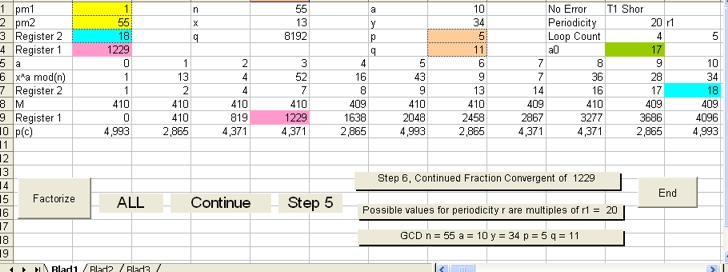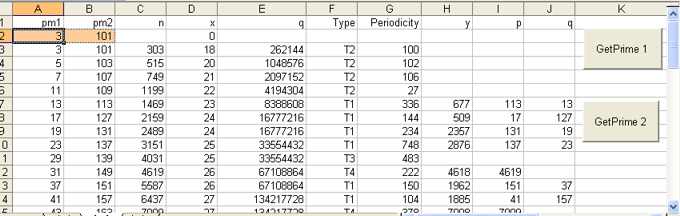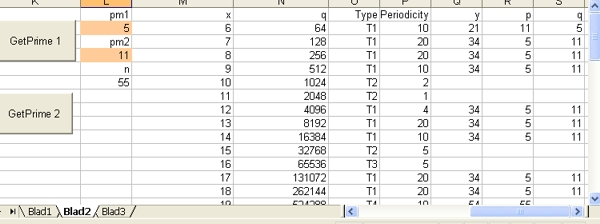## Shor's Algorithm Findprim.xls Operation

### Introduction and Purpose

This document descibes how to to operate the Excel Program Findprim.xls "Blad1" and "Blad2".
"Blad1" simulates Shor's Algorithm in 6 steps to factorize 1 number.
"Blad2" simulates Shor's Algorithm in 2 steps for 50 numbers.
For a copy of the Excel program in zip format select:FINDPRIM.XLS• "Blad1" Contains 2 Commands: Factorize and End
• The Command Factorize is used to start the program. After The Command is selected the Command will disappear. Go to the next paragragh for detailed description of the operation of the program.
• The Command End is used to end the program. In most cases this command is only used when it is not possible to continue. When the End is selected the Factorize is displayed and you can restart the program.
• "Blad1" also contains three selection controls . The text of the controls can change. They are explained in the next paragraph.
• "Blad1" also contains three Messages . The messages explain what to do or the results of the simulation.

1. In order to start the program select the Factorize Command
2. Input for the program are two (prime) numbers: pm1 and pm2. Multiplication of those two (prime) numbers gives a number n which is factorized using Shor's Algorithm. Those two numbers should be entered in the cells (1,B) and (2,B).
There are two ways to run the simulation:
• In 2 Steps. Input for the program are then two prime numbers.
For example if you want to factorize 55 in 2 Steps, you should enter the numbers 5 and 11.
• In 6 Steps. Input for the program are then two numbers. One number should be 1.
For example if you want to factorize 55 in 6 Steps, you should enter the numbers 1 and 55.
Shor's Algorithm simulation also works for two prime numbers:
For example: You can try the numbers 1 and 11
For a description of all those 6 Steps see: http://www.uwyo.edu/moorhouse/slides/talk2.pdf
In this paragraph the example of page 18 is followed. That means you should enter the numbers 1 and 55 and select the Continue Control
3. Next, as part of Step 1 the program displays the three calculated values n = pm1*pm2 in Cell (1,E) x in Cell (2,E) and q in Cell (3,E).
The values of q and x are calculated using the condition: 2n^2 < q = 2^x < 3n^2
The program displays the following Message: Option Enter x"
You can Enter a new value for x, if you want. If you do than a new value for q is calculated.
Select the Continue Control
4. As part of Step 2 the results of x^a mod n is calculated in row 6 as a function of a, with a going from 0 to q - 1. The value of a is displayed in row 5.
It is important to observe that the values in row 6repeats it self.
In this partical case (n = 55) the first number is a 1. The same value is at column "V", "AP", "BJ" and "CD". That means the same number 1 is in the columns 2, 22, 42, 62 and 82. In short the periodicity r is 20

What is important is the number 34 in row 6 in column "L". This number is exactly in the middle between the two number 1 in the columns 2 and 22. The number 34 is important in Step 6 of Shor's Algorithm. For more detail see: Shor's Algorithm - Answer Question 2

As part of step 2 an array of numbers is calculated how often each of the values (x^a mod n) is calculated. The values x^a mod n are displayed in row 7 and the array of numbers (the variable M) in row 8. In the program this array is called nxn(), explained in the next paragraph.
This means that row 6 and row 7 contain the same values. row 7 is also called Register 2 Select the Continue Control

5. Step 3 is called Measure Register 2 and the following message is displayed: Select a number from Register 2 line
There are two ways to do that:
• Random. In that case the program selects a random number out of the numbers displayed in Register 2 . If you want that to happen select Random
• User controlled. In that case select your self a number out of row 7 and select Select
In this particular case, because you want to find the prime numbers from composite number n=55:
Select in row 7 the number 28 (This is column "N") and select Select
6. Step 4 starts with the following message: Discrete Fourier Transformation in Register 1
Together with this Message two selection controls are dispalyed: Continue and Step 3
If you want to repeat the previous step: Select Step 3
In this particular case, select: Continue
7. Next as part of step 4 the following message is displayed: Observe FFT state values? together with three selection controls: ALL, Short or Skip
• When you select ALL all the FFT state values are displayed in "Blad 3"
• When you select Short than only for those values c the FFT state value is displayed when this number is larger than the square root of M.
• When you select Skip there is no display
What the display shows that the largest values are displayed in clusters.
In this particular case of n = 55 select: Skip
8. Next as part of step 4 the following message is displayed: Observe probability values? together with three selection controls: ALL, Short or Skip
• When you select ALL all the FFT state values are displayed in "Blad 3"
• When you select Short than only for those values c are displayed when p(c) is larger than 1/r
• When you select Skip there is no display
What the display shows that the most probable values displayed again are in clusters.
In this particular case of n = 55 select: Short
And compare the result with the display on page 20 in the above mentioned url. Called: "Step 5: Measure Register 1"
In row 20, 25, 30 and 35 in row B you have the higest probility values of 5.005.
In row 21 (for c = 410) and in row 24 (for c = 1638) you have the higest probability values of 2.863. Those 2 values repeat itself four times. Those same values are also on the display of page 20
In row 22 (for c = 819) and in row 23 (for c = 1229) you have the higest probability values of 4.379. Those 2 values repeat itself four times. Those same values are also on the display of page 20
What this means is that the results of the simulation are in accordance with the above mentioned url.
However not completly. The four higest values for c = 0, 2048, 4096 and 6144 do not match.
9. Next as part of step 4 the two messages "Grand probility total" and "Probability total " are displayed which each a value. In the case of n=55 the values are 100 and 96.17
The value of 100 means that the sum of all the probability values is 100%. This is as expected, which also indicates that the four values of 5.005 are correct.
This terminates step 4. Select Continue
10. Step 5 starts with the following message: Measure Register 1
Row 9 shows the same highest c values as displayed in red in the previous short list. This is Register 1
Row 10 shows the corresponding probability values
Row 11 shows periodicity values which are explained later on
Together with the above message the following message is displayed:
Select a number from Register 1 line
There are two ways to do that:
• Random. In that case the program selects a random number out of the numbers displayed in Register 1 . If you want that to happen select Random
• User controlled. In that case there are three options:
• You can select self a number out of row 9 and select Select
• In case there is a short list displayed starting from row 20, you can select one of those values and select Select
• You can select a value either way, modify the value and select Select. The purpose is to see what happens what if values with smaller probabilities are selected.
In this particular case select in row 9 the value 4915 and select Select
11. Step 6 starts with the following 3 messages:
• Continued Fraction Convergent of 4915 . The value 4915 is the value selected at the end of Step 5
• In this particular case: Possible values for periodicity r are multiples of r1 = 5
That means that possible periodicity values are 5, 10, 15, 20, 25 etc.
You now have to perform the following calculations:
13^5 mod 55 = 43 13^10 Mod 55 = 34 13^15 Mod 55 = 13 and 13^20 mod 55 = 1
The last calculation means that periodicity is 20, that a = 10 and that y = 34.
Finaly you have two perform two calculations:
• GCD (y-1,n) = GCD(33,55) = 11
• GCD (y+1,n) = GCD(35,55) = 5
• In this particular case: GCD n = 55 a = 10 y = 34 p = 5 q = 11
The Debug Buffer shows the following text:
 ``` 0 a = 0 p = 4915 / 8192 1 a = 1 p = 3277 / 4915 Convergent 1 / 1 2 a = 1 p = 1638 / 3277 Convergent 1 / 2 3 a = 2 p = 1 / 1638 Convergent 3 / 5 4 a = 1638 p = 0 / 1 Convergent 4915 / 8192 ```
Together with this three Message two selection controls are dispalyed: Continue and Step 5
If you want to repeat the previous step: Select Step 5
In this particular case, select: Continue For details see document 1 mentioned above.
12. At the end the following message is displayed This terminates Shor's factorization in 6 steps

Finally the factorization results of Classical Algorithm and Fermat's sieve Algorithm are displayed in resp. row 12 and row 13. In this case the Classical Algorithm requires 3 calculations Fermat's sieve Algorithm requires 1 Calculation.
For Example: try 5 and 43 to observe that Shor's Algorithm has periodicity 84, Classical Algorithm requires 4 calculations and Fermat's Algorithm requires 10 calculations.### Program Operation: "Blad2" GetPrime 1

The Purpose of the program is to calculate the two prime numbers pm1 and pm2 as a function of n. That means for different prime number combinations of pm1 and pm2.
The program displays the type (T1,T2,T3 or T4) and the periodicity as a function of each n.

Input for the program are two (prime) numbers: pm1 and pm2. Those two numbers should be entered in the cells (2,A) and (2,B). After that select the button "GetPrime 1"

Those two numbers are used as start values for 50 prime number combinations. Multiplication of those two numbers gives a number n which is factorized 50 times using Shor's Algorithm in 2 steps.

As part of Step 1 for each of the 50 combinations the program displays the three calculated values n = pm1*pm2 in Cell (3,C) x in Cell (3,D) and q in Cell (3,E). q = 2^x

As part of Step 2 for each of the 50 combinations x^a mod n is calculated as a function of a. This calculation terminates when the result is 1 or is equal to any previous calculated value.
When the result is 1 the periodicity is equal to a. This value is stored in Cell (3,G).

When the periodicity is even the calculation is of Type 1
When the periodicity is odd the calculation is of Type 3. In that case Shor's Algorithm does not work.
When the caculation detects any previous result not equal to 1 the calculation is of Type 2
Type is stored in Cell (3,E) as T1, T2 or T3.
For more detail goto Shor's Algorithm Answer Question 2.

What the program shows is that the periodicity becomes very large and in worst cases is almost equal to: pm1*pm2/2. This challenges the usefulness of Shor's Algorithm.

### "Blad2" GetPrime 1 - Example 1

In order to understand the functionality of GetPrime 1 try the following three examples:
1. Enter: for pm1 = 3 and for pm2 = 5 and select the Button GetPrime 1
In this particulair case n is a combination between two adjacent prime numbers. Observe that most of factorizations are of type T1.
The last two columns show the number of calculations (loops) to perform
• in case when factorization of the number n is used using Fermats factorization algorithm. That is why the text: Fermat
• as part of Modulair Exponentiation.. Modulair Exponentiation is involved as part of Step 5 and Step 6 of Shor's Agotithm. Modulair Exponentiation involves Right-to-left binary method That is why the text: Binary. For more detail select: Shor's Algorithm Reflection 4 - Modulair Exponentiation.
In this particular case all the values in the column Fermat are one and in column Binary are higher.
That means Fermats factorization algorithm out performs Shors Algorithm.
2. Next try the prime numbers pm1 = 100 and pm2 = 200.
The fact that both numbers are not important because the program first calculates the two nearest prime numbers.
Observe that in all cases Fermats factorization algorithm out performs Right-to-left binary method
3. The program also works for rather large prime number combinations.
For example: try 1000 and 4000.
The largest periodicity value is 2000000. Observe that in all cases Right-to-left binary method out performs Fermats factorization algorithm

### "Blad2" GetPrime 1 - Example 2 n=283321

In order to understand the functionality of GetPrime 1 in more detail try the following example:
Enter: for pm1 = 300 and for pm2 = 900 and select the Button GetPrime 1
The fact that both numbers are not important because the program first calculates the two nearest prime numbers. Observe line 4. This is the case when n=283321 and the two prime numbers are 311 and 911.
The number n is in this case of type 1 (this is a perfect example). That means that the number of values between the two stop signs (the number 1) is odd. That means that both the periodicity and the value y are easy to measure and or to calculate. For more detail goto Shor's Algorithm Answer Question 2.

The last two columns show the number of calculations (loops) to perform
• The coloumn Fermat indicates the number loops to perform when factorization of the number n is done, using Fermats factorization algorithm.
• The column Binary indicates Right-to-left binary method. The "Right-to-left binary method" is a part of Modulair Exponentiation.. Modulair Exponentiation is involved as part of Step 5 and Step 6 of Shor's Agotithm.
For more detail select: Shor's Algorithm Reflection 4 - Modulair Exponentiation.
In this particular case the two values in the columns Fermat and Binary are 79 and 15. That means Right-to-left binary method is the best and Shors Algorithm wins.
However it is not so simple as that. The number 15 is only valid when the period 28210 is measured. In that case Modulair Exponentiation is only used once. This is true in 32% of the cases.
In the case when 4030 (or less) is measured (at least) 98 loops have to be performed. This is the case in 32% of the cases. In these cases Fermats factorization algorithm wins.

Observe line 40. This is the case when n=417589 and the two prime numbers are 409 and 1021.
The period is 408. The two values in the columns Fermat and Binary are 69 and 9.
408 is measured in 31% cases. 204 is measured in 16% cases.
In the case when 34 (or less) is measured (at least) 97 loops have to be performed. This is the case in 14% of the cases. Only in these cases Fermats factorization algorithm wins.

In the case when pm1=1000 and pm2=4000 in all cases Right-to-left binary method out performs Fermats factorization algorithm. However that does not mean that Shor's Algorithm implemented on a Quantum Computer also wins, because different hardware problems can be at stake. Study Shor's Algorithm 10 Questions### Program Operation: "Blad2" GetPrime 2

The Purpose of the program is to calculate the two prime numbers pm1 and pm2 for a fixed value of n as a function of x
The program displays the type (T1,T2,T3 or T4) and the periodicity as a function of x

Input for the program are two (prime) numbers: pm1 and pm2. Those two numbers should be entered in the cells (2,L) and (4,L). After that select the button "GetPrime 2"

Those two numbers are used as start values for 50 prime number combinations. Multiplication of those two numbers gives a number n which is factorized 50 times using Shor's Algorithm in 2 steps.

The application "GetPrime 2" is specific written to study the article Oversimplifying quantum factoring in Nature. In this document of 11 July 2013 implementation constraints of Shor's Algorithm are discussed.

### Feedback

None

Created:19 April 2003
Modified 25 July 2013